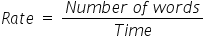Mathematics
Easy

Question

# Amra can type 400 words per hour. To finish writing a report containing 2000 words, she takes ________ hours.

## 5327Hint:

## The correct answer is: 5

### Amra types 400 words per hourWe have to find the rate at which Amra types. We will find it by taking the ratio of the number of words and the time required to type. As the rate is constant, we will use this value to find the number of hours required to type 2000 words.So the equation becomesSo the rate = 400 words per hour.Let’s find the time required to type 2000 words.We will rearrange this equation for time .For this, we will multiply both sides by time and divide by rate.Therefore, time required by Amra to type 2000 words is 5 hours.

We have to be careful about the units. We have to make sure that same quantities have same unit.

### Related Questions to study#### With Turito Foundation.#### Get an Expert Advice From Turito.